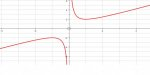# Range of y=x+1/x

#### apple2357

##### Junior Member
I am trying to work out the range of f(x) = x + 1/x, after sketching it, i notice i can use calculus to find the turning points
and establish it that way:But are there methods i can use that do not involve calculus? I am working with my son who hasn't met Calculus so i am trying to establish alternative approaches. I thought about guessing values and trying either side but that feels like you already know what the answer is? Unless someone can convince me this method is ok to do.

#### Subhotosh Khan

##### Super Moderator
Staff member
I am trying to work out the range of f(x) = x + 1/x, after sketching it, i notice i can use calculus to find the turning points
and establish it that way:View attachment 10852

But are there methods i can use that do not involve calculus? I am working with my son who hasn't met Calculus so i am trying to establish alternative approaches. I thought about guessing values and trying either side but that feels like you already know what the answer is? Unless someone can convince me this method is ok to do.
In my opinion, the easiest way to "approximate" maxima and minima (turning points) - without calculus - would be to plot the function (most of the time).

#### Dr.Peterson

##### Elite Member
I am trying to work out the range of f(x) = x + 1/x, after sketching it, i notice i can use calculus to find the turning points
and establish it that way:View attachment 10852

But are there methods i can use that do not involve calculus? I am working with my son who hasn't met Calculus so i am trying to establish alternative approaches. I thought about guessing values and trying either side but that feels like you already know what the answer is? Unless someone can convince me this method is ok to do.
One method is to try solving for x, and determine for what values of y it is possible. This is equivalent to finding the domain of the inverse function.

What happens here if you solve for x?

#### apple2357

##### Junior Member
One method is to try solving for x, and determine for what values of y it is possible. This is equivalent to finding the domain of the inverse function.

What happens here if you solve for x?
Ah yes, I'd forgotten that.
But finding the inverse is not easy, x+1/x= y , x^2+1= yx
x^2-yx +1=0 , (x-y/2)^2= y^2/4 -1
so inverse is y/2+-sqrt (0.25y^2-1) etc.
Doesn't look very pretty , but you are right it doesn't use calculus!

#### Dr.Peterson

##### Elite Member
Ah yes, I'd forgotten that.
But finding the inverse is not easy, x+1/x= y , x^2+1= yx
x^2-yx +1=0 , (x-y/2)^2= y^2/4 -1
so inverse is y/2+-sqrt (0.25y^2-1) etc.
Doesn't look very pretty , but you are right it doesn't use calculus!
It doesn't have to be pretty; but it's a little nicer if you use the quadratic formula, or clear fractions before taking the root.

And you really only needed the discriminant to find the range.

#### Otis

##### Senior Member
… inverse is y/2 +- sqrt(0.25y^2 - 1) … Doesn't look very pretty
I factored 1/4 from the radicand, and then simplified the sqrt(1/4) part.

x = y/2 ± 1/2∙√(y^2 - 4)

That's not so bad looking, for finding the domain of y (i.e., the range of y in the given function).

Last edited: